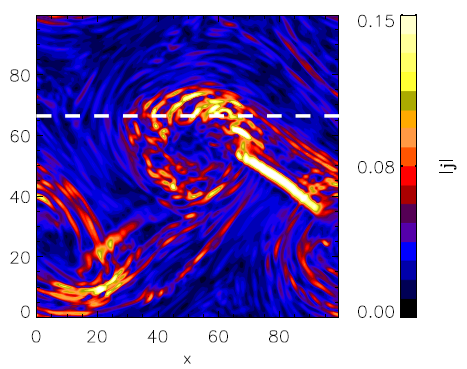## UNICAL_KH_2D

The numerical simulation has been performed by means of the Hybrid Vlasov Maxwell (HVM) code, in which protons are considered as kinetic species, while electrons are treated as a massless fluid.

The HVM system of equation has been integrated in a 5D phase space configuration (2D in physical space and 3D in velocity space). The aim of the present work is to study the development of the Kelvin-Helmholtz instability in presence of a uniform magnetic field perpendicular to the shear flow. Such a configuration is ubiquitous in ordinary fluids and plasmas. It can be representative of the region across the Earth’s magnetopause and has been indeed frequently detected by the MMS spacecraft.

Code equations are written in normalized units, with time scaled by the inverse proton cyclotron frequency ωcp , velocities by the Alfvén speed vA=B0/√(4πn0mp), (where B0 is the background magnetic field, n0 the density far from the shear, mp the proton mass), lengths by the proton skin depth dp = vAcp , the magnetic field by B0 and the density by n0 .Spatial domain D = [0, L] x [0, L] (L = 100 dp) is discretized on a uniformly spaced grid with Nx = Ny = 256  grid points; periodic boundary conditions have been implemented in the spatial domain. The velocity-space domain is discretized on a uniform grid with Nv,j = 71 (j = x, y, z)  grid points in each direction. Vanishing boundary conditions have been implemented: f(∣ vj ∣ > vmax) = 0 , where vmax = 7vth  and vth=T01/2  is the proton thermal speed in normalized units.

The main goals of this work is to analyze kinetic effects at proton scales in the nonlinear and turbulent stage of the Kelvin-Helmholtz instability in magnetized collisionless plasmas, and to point out the back reaction on particles triggered by the evolution of such instability, as energy reaches kinetic scales along the turbulent cascade.

An example of the turbulent 2D configuration with the development of the instability is depicted by the contour plot of the current density in the (x,y) plane (figure above). The white dashed line indicates the virtual satellite trajectory that can be found in the high-level data directory.

As a consequence of the interaction of particles with the turbulent fluctuating fields, the proton velocity distribution deviates significantly from the local thermodynamic equilibrium. An example of the proton VDFs is reported in the figures below, which show a highly distorted VDF and the presence of both anisotropic and agyrotropic features. The latter, even more evident in the cut plot (right panel).